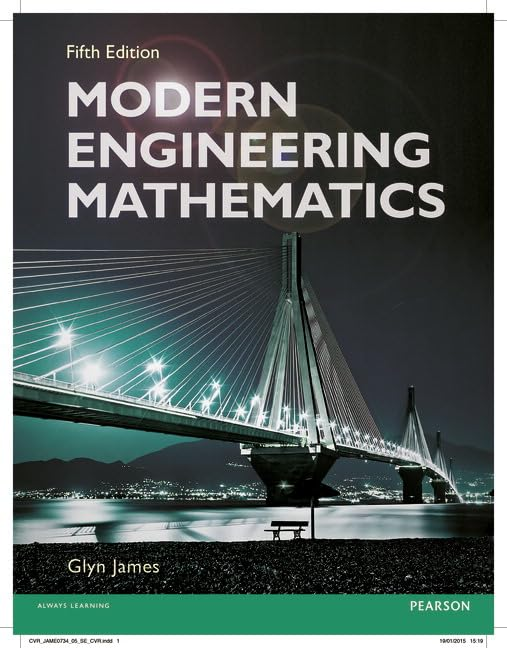# Modern Engineering Mathematics by Glyn James

Out of Stock
\$67.99
+10% GST
N/A
Only 0 left

## Modern Engineering Mathematics Summary

### Modern Engineering Mathematics by Glyn James

This book provides a complete course for first-year engineering mathematics. Whichever field of engineering you are studying, you will be most likely to require knowledge of the mathematics presented in this textbook. Taking a thorough approach, the authors put the concepts into an engineering context, so you can understand the relevance of mathematical techniques presented and gain a fuller appreciation of how to draw upon them throughout your studies.

### Why buy from World of BooksOur excellent value books literally don't cost the earthFree delivery in AustraliaEvery used book bought is one saved from landfill

Glyn James is currently Emeritus Professor in Mathematics at Coventry University, having previously been Dean of the School of Mathematical and Information Sciences. As in previous editions he has drawn upon the knowledge and experience of his co-authors to provide an excellent revision of the book.

Chapter 1: Numbers, Algebra and Geometry

1.1 Introduction

1.2 Number and arithmetic

1.3 Algebra

1.4 Geometry

1.5 Numbers and accuracy

1.6 Engineering applications

1.7 Review exercises

Chapter 2: Functions

2.1 Introduction

2.2 Basic definitions

2.4 Polynomial functions

2.5 Rational functions

2.6 Circular functions

2.7 Exponential, logarithmic and hyperbolic functions

2.8 Irrational functions

2.9 Numerical evaluation of functions

2.10 Engineering application: a design problem

2.11 Review exercises

Chapter 3: Complex Numbers

3.1 Introduction

3.2 Properties

3.3 Powers of complex numbers

3.4 Loci in the complex plane

3.5 Functions of a complex variable

3.6 Engineering application: alternating currents in electrical networks

3.7 Review exercises

Chapter 4: Vector Algebra

4.1 Introduction

4.2 Basic definitions and results

4.3 The vector treatment of the geometry of lines and planes

4.4 Engineering application: spin-dryer suspension

4.5 Engineering application: cable stayed bridge

4.6 Review exercises

Chapter 5: Matrix Algebra

5.1 Introduction

5.2 Definitions and properties

5.3 Determinants

5.4 The inverse matrix

5.5 Linear equations

5.6 Rank

5.7 The eigenvalue problem

5.8 Engineering application: spring systems

5.9 Engineering application: steady heat transfer through composite materials

5.10 Review exercises

Chapter 6: An Introduction to Discrete Mathematics

6.1 Introduction

6.2 Set theory

6.3 Switching and logic circuits

6.4 Propositional logic and methods of proof

6.5 Engineering application: expert systems

6.6 Engineering application: control

6.7 Review exercises

Chapter 7: Sequences, Series and Limits

7.1 Introduction

7.2 Sequences and series

7.3 Finite sequences and series

7.4 Recurrence relations

7.5 Limit of a sequence

7.6 Infinite series

7.7 Power series

7.8 Functions of a real variable

7.9 Continuity of functions of a real variable

7.10 Engineering application: insulator chain

7.11 Engineering application: approximating functions and Pade approximants

7.12 Review exercises

Chapter 8: Differentiation and Integration

8.1 Introduction

8.2 Differentiation

8.3 Techniques of differentiation

8.4 Higher derivatives

8.5 Applications of optimization problems

8.6 Numerical differentiation

8.7 Integration

8.8 Techniques of integration

8.9 Applications of integration

8.10 Numerical evaluation of integrals

8.11 Engineering application: design of prismatic channels

8.12 Engineering application: harmonic analysis of periodic functions

8.13 Review exercises

Chapter 9: Further Calculus

9.1 Introduction

9.2 Improper integrals

9.3 Some theorems with applications to numerical methods

9.4 Taylor's theorem and related results

9.5 Calculus of vectors

9.6 Functions of several variables

9.7 Taylor's theorem for functions of two variables

9.8 Engineering application: deflection of built-in column

9.9 Engineering application: streamlines in fluid dynamics

9.10 Review exercises

Chapter 10: Introduction to Ordinary Differential Equations

10.1 Introduction

10.2 Engineering examples

10.3 The classification of differential equations

10.4 Solving differential equations

10.5 First-order ordinary differential equations

10.6 Numerical solution of first-order ordinary differential equations

10.7 Engineering application: analysis of damper performance

10.8 Linear differential equations

10.9 Linear constant-coefficient differential equations

10.10 Engineering application: second-order linear constant-coefficient differential equations

10.11 Numerical solution of second-and higher-order differential equations

10.12 Qualitative analysis of second-order differential equations

10.13 Review exercises

Chapter 11: Introduction to Laplace Transforms

11.1 Introduction

11.2 The Laplace transform

11.3 Solution of differential equations

11.4 Engineering applications: electrical circuits and mechanical vibrations

11.5 Review exercises

Chapter 12: Introduction to Fourier Series

12.1 Introduction

12.2 Fourier series expansion

12.3 Functions defined over a finite interval

12.4 Differentiation and integration of Fourier series

12.5 Engineering application: analysis of a slider-crank mechanism

12.6 Review exercises

Chapter 13: Data Handling and Probability Theory

13.1 Introduction

13.2 The raw material of statistics

13.3 Probabilities of random events

13.4 Random variables

13.5 Important practical distributions

13.6 Engineering application: quality control

13.7 Engineering application: clustering of rare events

13.8 Review exercises

Appendix I Tables

Index

GOR008603929
Modern Engineering Mathematics by Glyn James
Glyn James